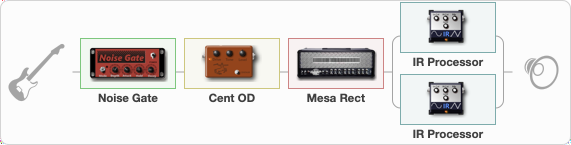# Megadeth - Countdown To Extinction

Discussion in 'ToneLib-GFX presets' started by grigoriy0192873, Mar 20, 2023.

1. ### grigoriy0192873Active Member

Preset name: Countdown To Extinction

Effects chain:Effect: "Noise Gate" (Dynamics / Filter), active - "yes"
{
"Mode" = Manual
"Depth" = 50
"Threshold" = 50
"Attack" = 0
"Hold" = 5
"Decay" = 250
}

Effect: "Cent OD" (Overdrive / Distortion), active - "yes"
{
"Drive" = 38
"Tone" = 76
"Level" = 38
}

Effect: "Mesa Rect" (Amp simulators), active - "yes"
{
"Gain" = 50
"Bass" = 50
"Middle" = 50
"Treble" = 50
"Presence" = 50
"Master" = 50
"Level (dB)" = 12
}

Effect: "Splitter" (Dynamics / Filter)
{
"A-Bypass" = Off
"A-Pan" = -25
"A-Level" = 55
"B-Bypass" = Off
"B-Pan" = 25
"B-Level" = 55
"Width" = 0

'A' branch:
{

Effect: "IR Processor" (Cabinets), active - "yes"
{
"Low Cut (Hz)" = 250
"Hi Cut (kHz)" = 20.0
"Mix" = 100
"Level (dB)" = 0
}
}
'B' branch:
{

Effect: "IR Processor" (Cabinets), active - "yes"
{
"Low Cut (Hz)" = 250
"Hi Cut (kHz)" = 20.0
"Mix" = 100
"Level (dB)" = 0
}
}
}

Note: You will need to download and install the ToneLib-GFX software to use the preset.